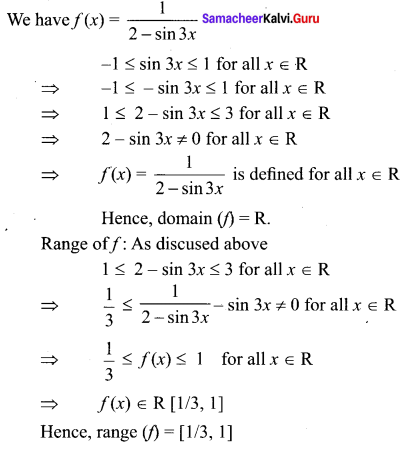Samacheer Kalvi 11th Maths Solutions Chapter 1 Sets Ex 1.3

Tamilnadu Samacheer Kalvi 11th Maths Solutions Chapter 1 Sets Ex 1.3

11th Maths Exercise 1.3 Answers Question 1.
Suppose that 120 students are studying in 4 sections of eleventh standard in a school. Let A denote the set of students and B denote the set of the sections. Define a relation from A to B as “x related toy if the student x belongs to the section y”. Is this relation a function? What can you say about the inverse relation? Explain your answer.
Solution:
(i) A = {set of students in 11th standard}
B = {set of sections in 11sup>th standard}
R : A ➝ B ⇒ x related to y
⇒ Every students in eleventh Standard must in one section of the eleventh standard.
⇒ It is a function.
Inverse relation cannot be a function since every section of eleventh standard cannot be related to one student in eleventh standard.

11th Maths Exercise 1.3 Answers Samacheer Question 2.
Write the values of f at – 4, 1, -2, 7, 0 if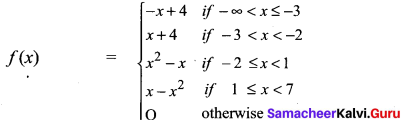Solution:
f(-4) = -(-4) + 4 = 8
f(1) = 1 – 12 = 0
f(-2) = (-2)2 – (-2) = 4 + 2 = 6
f(7) = 0
f(0) = 0

11th Maths Exercise 1.3 Question 3.
Write the values of f at -3, 5, 2, -1, 0 if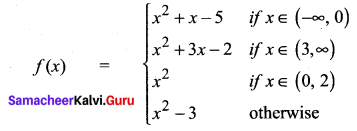Solution:
f(-3) = (-3)2 – 3 – 5 = 9 – 8 = 1
f(5) = (5)2 + 3(5) – 2 = 25 + 15 – 2 = 38
f(2) = 4 – 3 = 1
f(-1) = (-1)2 + (-1) – 5 = 1 – 6 = -5
f(0) = 0 – 3 = -3

11th Maths Exercise 1.3 Solutions Question 4.
State whether the following relations are functions or not. If it is a function check for one-to-oneness and ontoness. If it is not a function, state why?
(i) If A = {a, b, c] and/= {(a, c), (b, c), (c, b)};(f: A ➝ A).
(ii) If X = {x, y, z} and/= {(x, y), (x, z), (z, x)}; (f: X ➝ X).
Solution:
(i) f : A ➝ A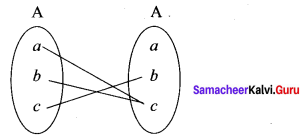It is a function but it is not 1 – 1 and not onto function.

(ii) f : X ➝ X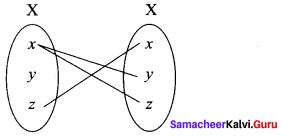x ∈ X (Domain) has two images in the co-domain x. It is not a function.

Exercise 1.3 Class 11 Maths State Board Question 5.
Let A = {1, 2, 3, 4} and B = {a, b, c, d}. Give a function from A ➝ B for each of the following:
(i) neither one-to-one nor onto.
(ii) not one-to-one but onto.
(iii) one-to-one but not onto.
(iv) one-to-one and onto.
Solution:
A = {1, 2, 3, 4}
B = {a, b, c, d}.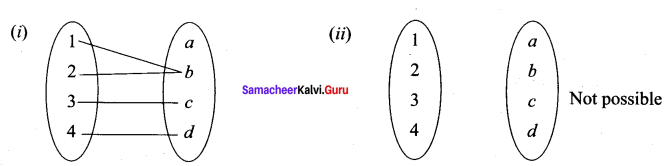R = {(1, b) (2, b) (3, c) (4, d)} is not 1-1 and not onto

(iii) Not possible

(iv)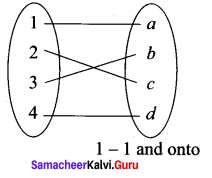11 Maths Exercise 1.3 Question 6.
Find the domain of $$\frac{1}{1-2 \sin x}$$
Solution: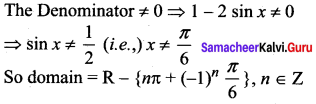11th Maths Exercise 1.3 In Tamil Question 7.
Find the largest possible domain of the real valued function f(x) = $$\frac{\sqrt{4-x^{2}}}{\sqrt{x^{2}-9}}$$
Solution: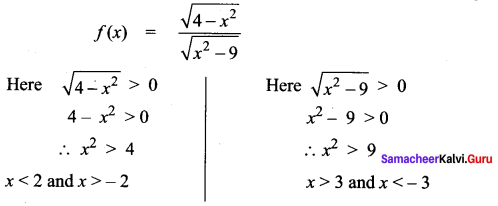∴ No largest possible domain
The domain is null set

Exercise 1.3 11th Maths Question 8.
Find the range of the function $$\frac{1}{2 \cos x-1}$$
Solution:
The range of cos x is – 1 to 1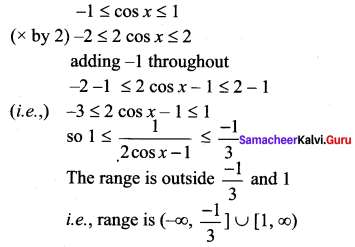11th Maths Exercise 1.3 10th Sum Question 9.
Show that the relation xy = -2 is a function for a suitable domain. Find the domain and the range of the function.
Solution:
xy = – 2 ⇒ y = -2/x
which is a function
The domain is (-∞, 0) ∪ (0, ∞) and range is R – {0}

Samacheer Kalvi Class 11 Maths Question 10.
If f, g : R ➝ R are defined by f(x) = |x| + x and g(x) = |x| – x, find gof and fog.
Solution: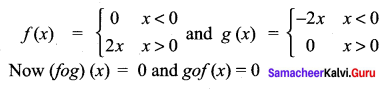Exercise 1.3 Class 11 Maths Question 11.
If f, g, h are real valued functions defined on R, then prove that
(f + g)oh = foh + goh. What can you say about fo(g + h) ? Justify your answer.
Solution:
Let f + g = k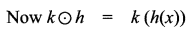= (f + g((h(x))
= f[h(x)] + g [h(x)]
= foh + goh
(i.e.,)(f + g)(o)h = foh + goh
fo(g + h) is also a function

Samacheer Kalvi Guru 11th Maths Question 12.
If f: R ➝ R is defined by f(x) = 3x – 5, prove that f is a bijection and find its inverse.
Solution:
P(x) = 3x – 5
Let y = 3x – 5 ⇒ 3x = y + 5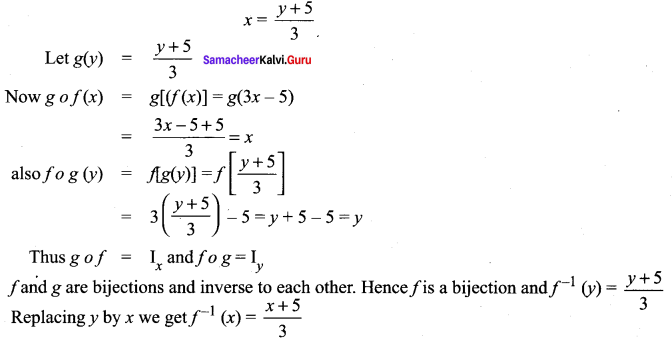Samacheer Kalvi 11th Maths Question 13.
The weight of the muscles of a man is a function of his body weight x and can be expressed as W(x) = 0.35x. Determine the domain of this function.
Solution:
W(x) = 0.35x
Since body weight x is positive and if it increases then W(x) also increase.
Domain is (0, ∞) i.e.,x > 0

Samacheer Kalvi.Guru 11th Maths Question 14.
The distance of an object falling is a function of time t and can be expressed as s(t) = -16t2. Graph the function and determine if it is one-to-one.
Solution:
s(t) = -16t2
Suppose S(t1) = S(t2)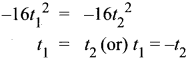since time cannot be negative, we to take t1 = t2
Hence it is one-one.

 t 0 1 2 3 s 0 -16 -64 -144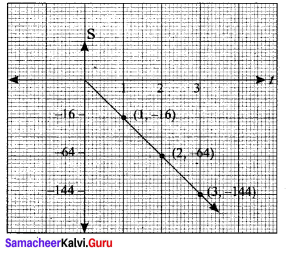Samacheer Kalvi Class 11 Maths Solutions Question 15.
The total cost of airfare on a given route is comprised of the base cost C and the fuel surcharge S in rupee. Both C and S are functions of the mileage m; C(m) = 0.4m + 50 and S(m) = 0.03m. Determine a function for the total cost of a ticket in terms of the mileage and find the airfare for flying 1600 miles.
Solution:
C – base cost,
S = fuel surcharge,
m = mileage
C(m) = 0.4 m + 50
S(m) = 0.03 m
Total cost = C(m) + S(m)
= 0.4 m + 50 + 0.03 m
= 0.43 m + 50
for 1600 miles
T(c) = 0.43 (1600) + 50 = 688 + 50 = ₹ 738

Samacheer Kalvi 11th Maths Solution Book Question 16.
A salesperson whose annual earnings can be represented by the function A(x) = 30, 000 + 0.04x, where x is the rupee value of the merchandise he sells. His son is also in sales and his earnings are represented by the function S(x) = 25, 000 + 0.05x. Find (A + S)(x) and determine the total family income if they each sell Rupees 1,50,00,000 worth of merchandise.
Solution:
A(x) = 30, 000 + 0.04x, where x is merchandise rupee value
S(x) = 25000 + 0.05 x
(A + S) (x) = A(x) + S(x)
= 30000 + 0.04x + 25000 + 0.05 x
= 55000 + 0.09x
(A + S) (x) = 55000+ 0.09x
They each sell x = 1,50,00,000 worth of merchandise
(A + S) x = 55000 + 0.09 (1,50,00,000)
= 55000 + 13,50,000
∴ Total income of family = ₹ 14,05,000

11th Maths Samacheer Kalvi Guru Question 17.
The function for exchanging American dollars for Singapore Dollar on a given day is f(x) = 1.23x, where x represents the number of American dollars. On the same day the function for exchanging Singapore Dollar to Indian Rupee is g(y) = 50.50y, where y represents the number of Singapore dollars. Write a function which will give the exchange rate of American dollars in terms of Indian rupee.
Solution:
f(x) = 1. 23x where x is number of American dollars.
g(y) = 50.50y where y is number of Singapore dollars.
gof(x) = g(f(x))
= g(1. 23x)
= 50.50 (1.23x)
= 62.115 x

Maths Class 11 Samacheer Kalvi Question 18.
The owner of a small restaurant can prepare a particular meal at a cost of Rupees 100. He estimates that if the menu price of the meal is x rupees, then the number of customers who will order that meal at that price in an evening is given by the function D(x) = 200 – x. Express his day revenue, total cost and profit on this meal as functions of x.
Solution:
cost of one meal = ₹ 100
Total cost = ₹ 100 (200 – x)
Number of customers = 200 – x
Day revenue = ₹ (200 – x) x
Total profit = day revenue – total cost
= (200 – x) x – (100) (200 – x)

Samacheer Kalvi Guru 11th Maths Solution Question 19.
The formula for converting from Fahrenheit to Celsius temperatures is $$y=\frac{5 x}{9}-\frac{160}{9}$$
Find the inverse of this function and determine whether the inverse is also a function.
Solution: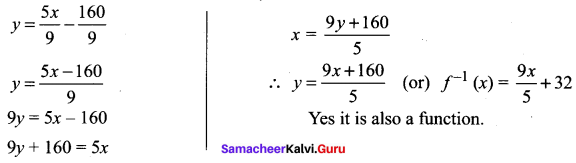Question 20.
A simple cipher takes a number and codes it, using the function f(x) = 3x – 4. Find the inverse of this function, determine whether the inverse is also a function and verify the symmetrical property about the line y = x (by drawing the lines).
Solution:
f(x) = 3x – 4
Let y = 3x – 4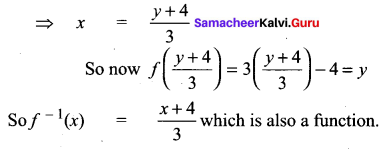Samacheer Kalvi 11th Maths Solutions Chapter 1 Sets Ex 1.3 Additional Questions

Question 1.
Find the domain and range of the function $$f(x)=\frac{1}{\sqrt{x-5}}$$
Solution:
Given that : f(x) $$f(x)=\frac{1}{\sqrt{x-5}}$$
Here, it is clear that / (x) is real when x – 5 > 0 ⇒ x > 5
Hence, the domain = (5, ∞)
Now to find the range put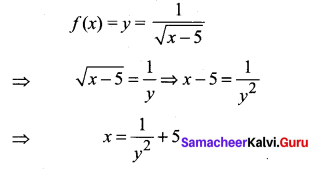For x ∈ (5, ∞), y ∈ R+.
Hence, the range of f = R+.

Question 2.
If $$f(x)=\frac{x-1}{x+1}$$, then show that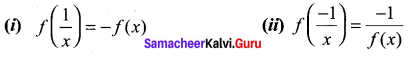Solution: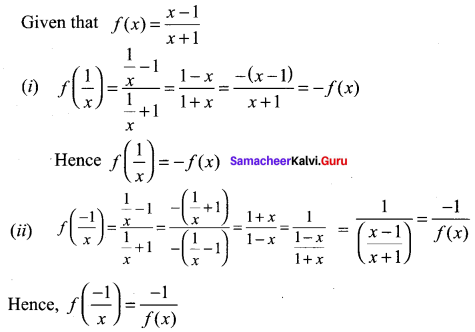Question 3.
Find the domain of each of the following functions given by:
$$f(x)=\frac{x^{3}-x+3}{x^{2}-1}$$
Solution: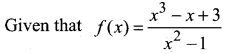Here, f(x) is not defined if x2 – 1 ≠ 0
(x – 1) (x + 1) ≠ 0
x ≠ 1, x ≠ -1
Hence, the domain of f = R – {-1, 1}

Question 4.
Find the range of the following functions given by
f(x) = 1 + 3 cos 2x
Solution:
Given that: f(x) = 1 + 3 cos 2x
We know that -1 ≤ cos 2x ≤ 1
⇒ -3 ≤ 3 cos 2x ≤ 3 ⇒ -3 + 1 ≤ 1 + 3 cos 2x ≤ 3 + 1
⇒ -2 ≤ 1 + 3 cos 2x ≤ 4 ⇒ -2 ≤ f(x) ≤ 4
Hence the range of f = [-2, 4]

Question 5.
Find the domain and range of the function $$f(x)=\frac{x^{2}-9}{x-3}$$
Solution: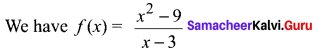Domain off: Clearly f(x) is not defined for x – 3 = 0 i.e. x = 3.
Therefore, Domain (f) = R – {3}
Range off: Let f(x) = y. Then,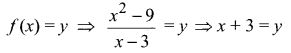It follows from the above relation that y takes all real values except 6 when x takes values in the set R – {3}. Therefore, Range (f) = R {6}.

Question 6.
Find the range of the following functions given by f(x) = $$\frac{1}{2-\sin 3 x}$$
Solution: